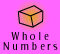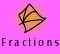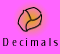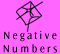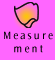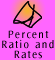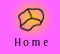| Ordering positive and negative numbers | Absolute Value | The unary negative sign and the binary subtraction sign |
| Using a calculator | Quick quiz |

Ordering positive and negative numbers

 If a number has a positive sign in front of it or no sign in front of it, it is a positive number. Convention says that we can choose to omit the positive sign from the front of a positive number; however when we are working with lots of positive and negative numbers it is a good idea to include the direction signs so that there is less chance of making an error.

Greater than and less than

We often use the greater than and less than signs to record comparison of numbers.

 less than < greater than > 4 < 10 5 > -2 4 is less than 10 5 is greater than -2

NOTE: To remember the difference between less than and greater than, remember that the smaller pointy end always points to the smaller number.

Example 1: Decide whether to use the > or < sign in the following examples.

 a) 4 _ 18 b) 9 _ -5 c) -7 _ -21 d) -46 _ -9

You may want to draw a number line to help.

 Working Out Thinking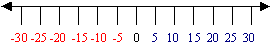a) 4 < 18, 4 is less than 18 b) 9 > -5, 9 is greater than -5 c) -7 > -21, -7 is greater than -21 d) -46 < -9, -46 is less than -9 I have drawn a quick number line with a very small scale, so I can only see every 5th integer marked. This scale is sufficient for me to use the number line to help answer these questions because I can approximate where each number lies on the number line.

Bigger or smaller

People often get confused when comparing negative numbers.

For example, which number is smaller -5 or -17?

You could draw a quick number line to remind you (as we have done above) or another easy way to think of this problem is to think of the numbers as temperatures. Which temperature is colder (lower), -5° C or -17° C?

Example 2: Arrange the following numbers in order from smallest to largest.

 -19, -3.5, 3, 0.7, 23, -7, 0, 1/4, 18, -2, -5, -0.3, 16, 8, -1/5, 21, -4, -23, 0.2

 Thinking First I'll scan the numbers and look for the smallest negative numbers. By this I mean the biggest numbers with negative signs because the bigger the number with the negative sign the further away from zero it will be. Then I'll gradually work my way towards zero and then scan the positive numbers for the smallest to largest numbers. (I can imagine working along a number line from negative infinity to positive infinity.) -23, -19, -7, -5, -4, -3.5, -2, -0.3, -1/5, 0, 0.2, 1/4, 0.7, 3, 8, 16, 18, 21, 23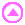Absolute Value

Absolute value is an alternative meaning of size.

You may not think it is sensible to say that a 'big' number like -16 is less than a 'small' number like -3. For example, more money is involved in a debt of \$16m than a debt of \$3m. A town 16 km south of where I am is further away from me than a town that is 3 km south.

This second idea of size is called 'absolute value'. The absolute value of -16 is +16 and is written |-16|. The absolute value of +16 is written |+16| and is just equal to 16. The absolute value of a number is the distance that it is from zero.

So -16 < -3 but |-16| > |-3| .

On the one hand -16 < -3 because -16 is to the left of -3 on the number line. Later, we will show that if we subtract -16 from -3, the answer is positive, which is the formal definition of one number being greater than another.

But |-16| > |-3| because 16 is further away from zero than 3. |-16| = 16 and |-3| = 3.

 There are two ideas of size. One refers to the left/right ordering of positions on the number line. The absolute value idea refers to the distance from zero.

Example 3:

Answer true or false to the following statements:

 a) -11 < -5 b) |-11| < |-5| c) -11 < |-5| d) |-11| < -5

 Working Out Thinking a) -11 < -5 True -11 is further below zero than is -5 b) |-11| < |-5| False -11 is further away from zero than is -5 c) -11 < |-5| True The absolute value of -5 is 5. -11 is less than 5. d) |-11| < -5 False The absolute value of -11 is 11. 11 is not less than -5.The unary negative sign and the binary subtraction sign

As we have mentioned a positive sign (or no sign) or a negative sign in front of a number indicates its direction from zero, i.e. that it is positive or negative. We can think of a number's direction as being an attribute or property of the number. A negative number has the property of being negative while a positive number has the property of being positive.

-4, +5, +8, -7, 12, -43, 560, -941

-4 is the number 4 with the property of being negative. We call it negative four.
12 is the number 12 with the property of being positive. We call it 12 or positive 12.

When we use a positive or negative sign in this sense we call it a unary sign because it applies to one number.

Unfortunately we also use the same symbols to mean subtraction or addition. When we use a sign in this sense we call it a binary sign because it signifies an operation connecting two numbers.

For example, 10 - 4 = 6

Here the symbol means 'subtract', 10 take away 4 equals 6.

The example below includes both negative numbers and subtraction.

For example, (-4) - 6 = (-10)

We read this example as "negative 4 subtract 6 equals negative 10".

The '-' sign in front of the 4 indicates that the 4 is negative while the '-' between -4 and 6 means subtract.

Confusion can arise because we use the same symbol to mean 2 different things. Some people also use the same terminology. We could have read the above example as minus 4 minus 6 equals minus 10 yet the word minus clearly has two different meanings. Minus means the number it is in front of has the property of being negative whereas minus means take away. This is not good practice.

For example, (-4) - (-6) = 2

Here we have three occurrences. The '-' in front of the 4 and 6 indicate that they are negative numbers and the '-' sign between the two numbers means 'subtract'. We read the example as 'negative 4 subtract negative 6 equals 2'.

To differentiate between the two meanings we often hand write the signs for positive and negative in a smaller font raised above the level of the text. This difference is not always maintained and cannot always be adhered to in printed or electronic documents. We are using brackets to make the meanings clear.

 4 - 2 4 - (- 2) 4 subtract 2 4 subtract negative 2

Using a calculator

You do not use the subtraction button on a calculator to enter a negative number. Calculators have separate buttons for the unary and binary sign.

Most simple calculators have a change of sign button, which enables you to enter positive and negative integers.

 +/-
 -
change of sign button
subtraction button

Example: Do you know how to type in the following numbers in your calculator?

 a) -7 b) - 54 c) -980

 a) 7 +/- screen display: -7 b) 54 +/- screen display: -54 c) 980 +/- screen display: -980

On most simple calculators, in order to indicate that a number is negative we must first key in the number and then change the sign of the number via the '+/-' key. You will notice that if you repeatedly press the '+/-' key the number displayed will alternate between being displayed as negative and positive. Experiment on your own calculator. What happens when you press the change of sign key when you have zero displayed on the screen?

Example: (-49) - 786

Press keys:

 49 +/- - 786 =

Example: -34 + (- 57) - (- 22)

Press keys:

 34 +/- + 57 +/- - 22 +/- =

For more information on using a calculator see Whole Numbers, Multiplication, Using a Calculator or Percent, Ratio and Rates, Percent Examples,Calculator Shortcuts.Quick quiz

1. Insert greater than (>) or less than (<) signs between each of the following sets of numbers.
a) (6) _ (-1)
b) (- 9) _ (-12)
c) (0) _ (-3)
d) (17) _ (-17)
e) (6) _ (-5) and (-5)_ (-22)
f) (-41) _ (8) and (8) _ (-2)
2. Order the following sets of numbers from smallest to largest .
a) -9, 3, 71, -7, 0, -1, 1, 0.5, -1/3
b) -6, 0.15, 12, -9, -12, 0, -3, -15
c) 4, 1/5, 0, -1.3, -31, -4, -157, -0.4
d) -419, -328, -2, 0, -125, 10, -325.36, 12.5, 12/15

3. Perform the following operations on a simple calculator, using
 +/-
button to change the sign where appropriate.
a) 45 - 79
b) 53 - (-78)
c) -34 + 9
d) (- 168) - 94
e) 67 - (-5) + -2
f) -19 + (-5) - 17 - 3

4. Put the following numbers on a number line.
0.22, -1.8, 0.17, -0.17, 1/17, -1/17, 1, 1 1/4, 1/4
5. Which is the greatest number?
a) (-5), (-4) or (-1)
b) (-10) ,(0) or (+0.1)
c) (-0.1), (-0.2) or (-1/2)